Function Repository Resource:

# PopulationVariance

Compute the population variance for a set of data

Contributed by: Wolfram|Alpha Math Team
 ResourceFunction["PopulationVariance"][{x1,x2,…}] gives the population variance of the given data.

## Details and Options

ResourceFunction["PopulationVariance"][list] is equivalent to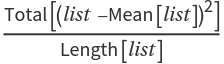for real-valued data.
ResourceFunction["PopulationVariance"] handles both numerical and symbolic data.

## Examples

### Basic Examples (3)

Compute the population variance of a list:

 In:=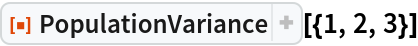Out=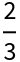Compute the population variance of a different list:

 In:=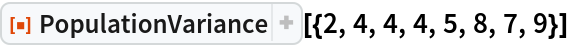Out=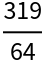Compute the population variance of another list:

 In:=Out=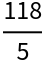Compare with the known expression for population variance as the average square difference to the mean:

 In:=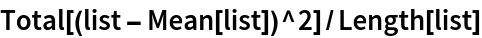Out=### Scope (1)

PopulationVariance works on both numeric and symbolic input:

 In:=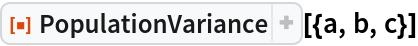Out=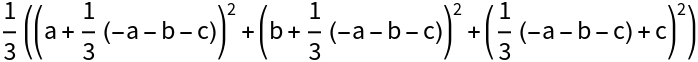### Properties and Relations (2)

The population variance of a data set differs from its sample variance calculated with Variance due to Bessel's correction:

 In:=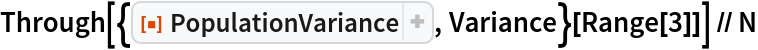Out=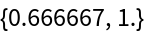The fractional effect of this correction tends to be smaller for larger sample sizes:

 In:=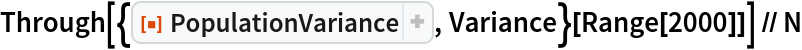Out=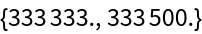PopulationVariance[…] is equivalent to ResourceFunction["PopulationStandardDeviation"][…]2:

 In:=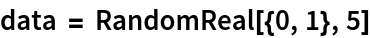Out=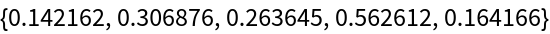In:=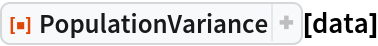Out=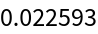In:=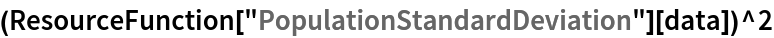Out=## Publisher

Wolfram|Alpha Math Team

## Version History

• 4.0.0 – 23 March 2023
• 3.1.0 – 11 May 2021
• 3.0.0 – 24 January 2020
• 2.0.0 – 06 September 2019
• 1.0.0 – 05 August 2019